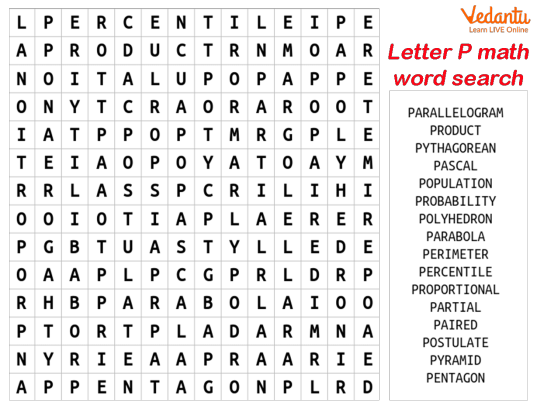Courses
Courses for Kids
Free study material
Free LIVE classes
MoreWhat is the first Maths term that comes to your mind when asked about any word that starts with P? Perpendicular, polygon, perimeter, and many more. We hear these terms every day while solving Maths problems. But do you know the definition of each and every term? If not, then be ready to learn about some important Maths terms today along with their definitions. The interesting fact is that all these terms start with the letter P. So, let us find out how many Maths words are there which start with P.## List of Maths Terms Starting with ‘P’

Given below are some of the important Mathematical terms starting with the letter P. Knowing these terms will help you to understand the concepts of Mathematics easily.

• Parallel lines: Lines that never intersect or cross one another on a plane.

• Parallelogram: A quadrilateral with two pairs of equal and parallel sides.

• Partial product: It is defined as the product(s) obtained during the intermediate stages in order to complete a multiplication process.

• Pentagon: A polygon with 5 sides.

• Percent: Part per one hundred.

• Percentage: The answer obtained after a number is multiplied by a percent.

• Perimeter: The distance around the outside of a closed figure.

• Perpendicular lines: Lines that form 4 right angles when they meet or intersect.

• Pi: The ratio of the perimeter of a circle to the diameter.

• Polygon: A closed geometric figure with at least 3 sides.

• Positive integer: A whole number bigger than 0.

• Power of ten: The product of multiplying ten by itself one or more times. For example, 105 is the product of multiplying 10 by itself 5 times. Thus, 105 = 10 × 10 × 10 × 10 × 10.

• Prime factorization: A number written as the product of its prime factors only.

• Prime number: A number that can be divided evenly only by one and the number itself.

• Principal: Amount borrowed or invested.

• Product: The answer to a multiplication problem.

• Proper fraction: A fraction with a smaller numerator than a denominator.

• Proportion: Two equal ratios.

• Protractor: A geometric instrument used to measure or draw angles.

• Pyramid: A solid figure that has triangular sides and a base that is a polygon.

## Conclusion

We hope that this list has helped you learn about some important Mathematical terms and their meaning. There are a lot more terms that start with the letter P. We advise you to go through these terms regularly to be familiar with their definitions and meanings.

Last updated date: 23rd Sep 2023
Total views: 136.2k
Views today: 2.36k